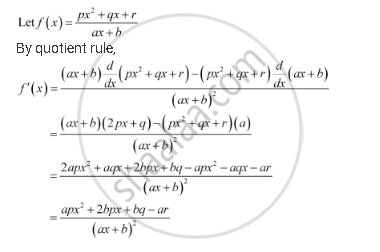CBSE (Arts) Class 11CBSE
Share

# Find the Derivative of the Following Functions (It is to Be Understood That A, B, C, D, P, Q, R And S Are Fixed Non-zero Constants And M And N Are Integers): (Px^2 +Qx + R)/(Ax +B) - CBSE (Arts) Class 11 - Mathematics

ConceptDerivative Algebra of Derivative of Functions

#### Question

Find the derivative of the following functions (it is to be understood that abcdp, q, r and s are fixed non-zero constants and m and n are integers): (px^2 +qx + r)/(ax +b)

#### SolutionIs there an error in this question or solution?

#### APPEARS IN

NCERT Solution for Mathematics Textbook for Class 11 (2018 to Current)
Chapter 13: Limits and Derivatives
Q: 9 | Page no. 317

#### Video TutorialsVIEW ALL 

Solution Find the Derivative of the Following Functions (It is to Be Understood That A, B, C, D, P, Q, R And S Are Fixed Non-zero Constants And M And N Are Integers): (Px^2 +Qx + R)/(Ax +B) Concept: Derivative - Algebra of Derivative of Functions.
S